Next: Newton's method for non-linear Up: Annexes Previous: The secant equation   Contents

# 1D Newton's search

Suppose we want to find the root of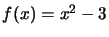(see Figure 13.4). If our current estimate of the answer is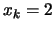, we can get a better estimate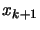by drawing the line that is tangent to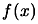at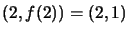, and find the pointwhere this line crosses the x axis. Since,and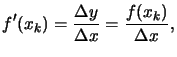we have that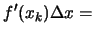F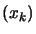or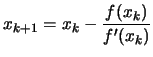(13.30)

which gives. We apply the same process and iterate on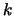.Frank Vanden Berghen 2004-04-19